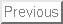Mathematics 23 Syllabus Boyce and DiPrima

The Undergraduate Program Committee

Date: May 12, 1999

Lecture Topics/Sections Some Standard Examples/Concepts
Day 1 2.5 - 2.6 Modeling: decay, mixing, cooling, growth. Physical models and the differential equations which result.
Day 2 2.1, 2.2, 2.3, 2.4 Review derivation of solution to first order linear, and review separable equations. Mixing problems (equal rate in/out is separable; unequal rate is FOL)
Day 3 5.2 Series solutions to first order linear or second order constant coefficient. Solve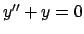via series, and isolate fundamental solutions as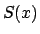and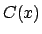. Observe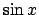and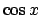are also solutions. How areandrelated toand? Leads to representation of functions by series.
Day 4   Define Taylor polynomials; Define geometric series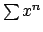and show when it converges to the rational function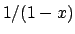; Discuss the notion of Taylor series, and the notion of an interval of convergence: examplesand.
Day 5   Define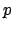-series and show when they convrge via an improper integral; state the comparison test and ratio test. Ratio test can be deduced from the comparison test. Use ration test to define the radius of convergence of a power series.
Day 6   Define the notion of absolute/conditional convergence; Discuss alternating series and the error resulting from using partial sums. Consider as an application, the evaluation of (sine, cosine,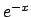). How could one build afunction for a calculator via partial sums of the Taylor series for the sine?
Day 7 3.1 - 3.3 Review of second order constant coefficient (real roots),
Just use characteristic equation and unmotivated solutions here (proofs in a couple of days)
Day 8 3.4 Review of complex numbers, complex exponential and second order constant coefficient (complex roots).
Day 9 11.3, 11.4 [Crowell & Slesnick] Linear Differential Operators (formal ring properties). Homogeneous and nonhomogeneous solutions
Set of homogeneous solutions forms a vector space
General solution of the form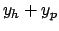Day 10 11.4 [Crowell & Slesnick] Solve second-order constant coefficient problem by reducing to a first-order system and using general solution to FOL equations.
Day 11 4.1, 3.3 Linear independence of solutions. General definition.
Theorem 4.12 [4.1] (w/o Wronskian), that is dimension of space of homog solutions equals the order of the equation. Detecting linear independence: the Wronskian
Higher dimensional case after a review of determinants
Day 12 7.2 Review of matrices, determinants (alternating nature)
Day 13 4.1 Wronskian (higher order): problem 20 page 207
Take an nth order linear DE and write it as a system. Introduce matrix notation for this motivating Chapter 7. Perhaps revisit Theorem 4.12.
Day 14 3.6 3.7 [Nonhomogeneous and nonconstant coefficient equations] Finding a particular solution. Undetermined coefficients and variation of parameters.
Day 15 3.8/7.1 Harmonic Oscillators (simple and not)
Day 16 7.3 Systems of linear equations, linear independence, eigenvalues and eigenvectors.
Day 17 7.3 Systems of linear equations, linear independence, eigenvalues and eigenvectors.
Day 18 7.4 Basic Theory of First order systems
Day 19 7.5 Homogeneous linear systems with constant coefficients
Day 20 7.6 Complex Eigenvalues
Day 21 7.7, 10.1 Repeated eigenvalues, Separation of Variables (heat conduction)
Day 22 10.1 Heat equation
Day 23 10.2 Fourier Series
Day 24 10.3, 10.4 Fourier Convergence Theorem; Even and odd functions
Day 25 10.4, 10.5 Even and odd functions; More general heat equation
Day 26 10.6 The Wave Equation
Day 27 10.7 Laplace's Equation
Day 28 Wrap it up